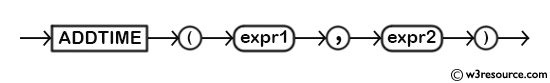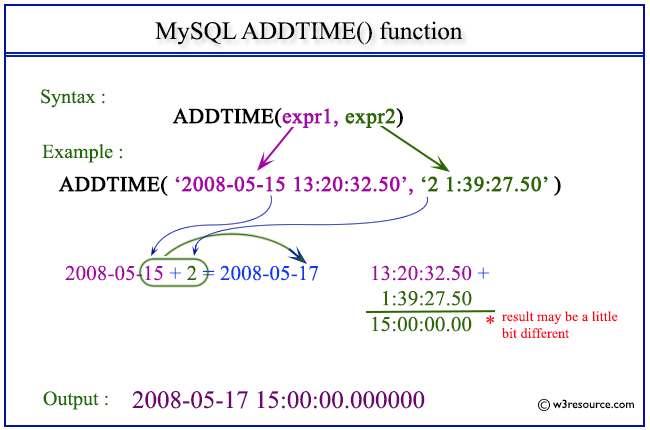The ADDTIME() function in MySQL is used to add a time interval to a given time value. Here are some examples of its application:

• Added time to a timestamp or datetime value: The ADDTIME() function can be used to add a time interval to a timestamp or datetime value. This can be useful when you need to adjust a timestamp or datetime value by a specific amount of time.
• Calculating the duration of an event: If we have a start and an end time for an event, we can use the ADDTIME() function to calculate the duration of the event. Subtract the start time from the end time to get the total elapsed time.

Syntax:

```ADDTIME(expr1,expr2)
```

Arguments:

Name Description
expr1 A datetime.
expr2 A time value.

Syntax Diagram:MySQL Version: 8.0The following statement will return a time after adding 2 days 1 hr. 39 min. and 27.50 sec. with the datetime 2008-05-15 13:20:32.50.

```SELECT ADDTIME('2008-05-15 13:20:32.50','2 1:39:27.50') as required_datetime; ```

Sample Output:

```mysql> SELECT ADDTIME('2008-05-15 13:20:32.50','2 1:39:27.50') as required_datetime;
+----------------------------+
| required_datetime          |
+----------------------------+
| 2008-05-17 15:00:00.000000 |
+----------------------------+
1 row in set (0.00 sec)
```

Example: ADDTIME() function using time value

The following statement will return a time after adding 1 hr. 39 min. and 27.50 sec. with the time 13:20:32.50.

`SELECT ADDTIME(‘13:20:32.50’,’1:39:27.50’) as required_datetime; `

Sample Output:

```mysql> SELECT ADDTIME('13:20:32.50','1:39:27.50') as required_datetime;
+-------------------+
| required_datetime |
+-------------------+
| 15:00:00.000000   |
+-------------------+
1 row in set (0.00 sec)
```

How to add a negative time interval to a time value using MySQL's ADDTIME() function?

We can add a negative time interval to a time value using the ADDTIME() function in MySQL. For example:

```SELECT ADDTIME('11:30:00', '-01:15:30'); ```

Sample Output:

```ADDTIME('11:30:00', '-01:15:30')
10:14:30```

In the above example, we subtract the time interval '01:15:30' from the original time value '11:30:00'. The result of this query will be '10:14:30', which is the difference between the two time values.

How to format the ADDTIME() function output in MySQL?

When using the ADDTIME() function in MySQL, the resulting date is returned in the default HH:MM:SS format. However, we can format the function output using the TIME_FORMAT() function. Here are some examples of how to format the ADDTIME() output in MySQL:

``````SELECT TIME_FORMAT(ADDTIME('11:30:00', '01:15:30'), '%H:%i:%s');
```
```

Sample Output:

```TIME_FORMAT(ADDTIME('11:30:00', '01:15:30'), '%H:%i:%s')
12:45:30
12:45 PM
12:45:30 PM
12:45:30 PM
```

How to use variables or expressions in MySQL's ADDTIME() function?

We can use variables or expressions in the ADDTIME() function in MySQL, as long as they evaluate to a valid time value.

Here's an example of using a variable as an input to the ADDTIME() function:

``````SET @time_val = '06:00:00';
SET @time_interval = '02:30:00';
```
```

Sample Output:

```ADDTIME(@time_val, @time_interval)
08:30:00```

All Date and Time Functions :